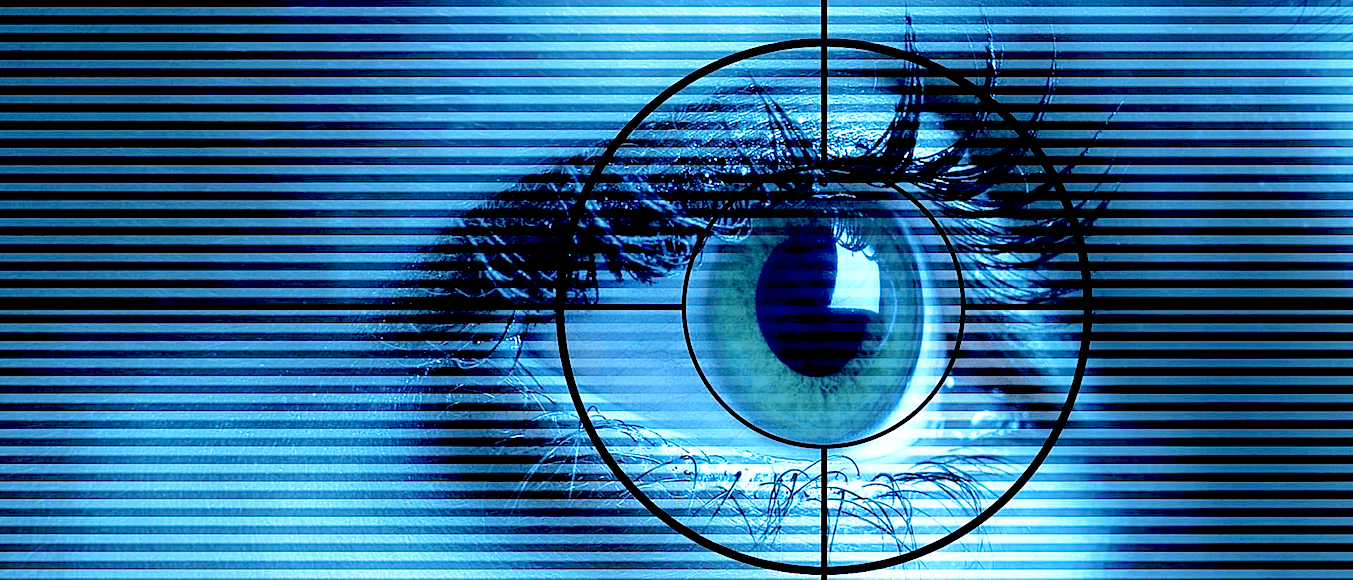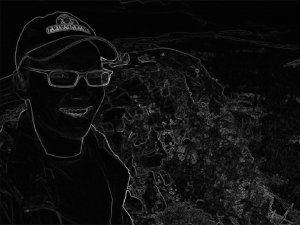# Finding the edge of your images

How can we identify objects in photos, to diagnose cancer or predict the weather? Using gradients!Vision is an important part of human perception. We use it on a daily basis: when crossing the road, for example, vision helps us to identify any oncoming dangers like cars or cyclists.

On top of this, how our vision perceives things is important when deciding how to present data or information to the user. How do we track down landmarks on a satellite map? How do we determine from a weather forecast whether it’s going to rain in a particular place? Or in medicine, how do we diagnose diseases from an x-ray scan?

Despite the wide applications, there is one fundamental idea. For any image, we need tools that enable us to give a meaningful description of what it represents. This is why finding edges can be really useful! It help us to distinguish one object from another.

### How does maths come into play?

Let’s keep it simple! We will only consider greyscale images. The most common way to mathematically represent a greyscale image is through a matrix, like the one below:

\begin{equation}
f_{x,y} =
\begin{pmatrix}
f_{1,1} & f_{1,2} & \cdots & f_{1,n} \\
f_{2,1} & f_{2,2} & \cdots & f_{2,n} \\
\vdots & \vdots & \ddots & \vdots \\
f_{m,1} & f_{m,2} & \cdots & f_{m,n}
\end{pmatrix}.
\end{equation}

Here, our matrix simply stores all the information we need to describe any greyscale image. Let’s just take any entry $e$, say $e = f_{i,j}$. The value of $e$ determines the brightness: the larger the $e$, the brighter the point. We also know the location of the brightness. In this case the brightness is on the $i^{\text{th}}$ row and on the $j^{\text{th}}$ column. Confused? Don’t worry, let’s look at some examples$\ldots$

Examples of greyscale images include:
A binary image\begin{equation}
f_{x,y} = \begin{pmatrix}
1 & 0 & 0\\
0 & 1 & 0\\
0 & 0 & 1
\end{pmatrix}
\end{equation}

A greyscale image\begin{equation}
f_{x,y} = \begin{pmatrix}
1 & 2 & 3\\
5 & 3 & 5\\
4 & 2 & 1
\end{pmatrix}
\end{equation}\begin{equation}
f_{x} = \begin{pmatrix}
1 & 2 & \ldots & 50
\end{pmatrix}
\end{equation}

### Edges in images

Our next question is this: how do we describe an edge in our image? Well, let’s look at this example:Edge

Clearly, we can see the edge in the middle of our image. Now let’s take all the values along a row on the image and plot them out from left to right.As you can see, there is a massive change in values in the middle of the graph. Since we measure the change in values by the gradient, we can say that an edge is where there is a large gradient in the image.

### The gradient of an image

In order to find the gradient we simply use this equation:
\begin{equation}
\mbox{ Gradient} = \left\{ ( \mbox{ $\Delta x$ of $f$ } ) ^2 + ( \mbox{ $\Delta y$ of $f$ } )^2 \right\} ^{1/2},
\end{equation}
where the $\Delta$ means ‘the change’.  This is called the Eikonal equation.

With the theory done, let’s now look at an example. Take this image where our resident chocolate fountain expert Adam is on holiday in Yosemite National Park:Original picture (B&W)

Now let’s apply our Eikonal equation to this image, to give:Here the brighter the pixel at a particular point in the image, the larger the gradient at that point. Hence in order to find the edges, we simply consider all the pixels that are above a fixed value. The fixed value is defined by the user, i.e. us! In most cases, it is chosen to lie in the highest 10% of all the values that are generated by the application of the Eikonal equation. To demonstrate, let us experiment with various thresholds:### Final words

Thus we have completed a quick guide to the basics of edge detection! One of the applications of edge detection is in image segmentation. Image segmentation simply means dividing an image into parts that contain a particular property. Image analysis give us an opportunity to apply very abstract maths in a practical setting.

### ReferencesKin Quan is a PhD student in the Centre for Medical Image Computing at University College London.